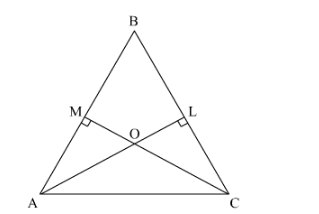# In ∆ABC, AL and CM are the perpendiculars from the vertices A and C to BC and AB respectively. If AL and CM intersect at O, prove that:

Question:

In ∆ABC, AL and CM are the perpendiculars from the vertices A and C to BC and AB respectively. If AL and CM intersect at O, prove that:

(i) $\triangle \mathrm{OMA} \sim \triangle \mathrm{OLC}$

(ii) $\mathrm{OAOC}=\mathrm{OMOL}$

Solution:i. In $\triangle O M A$ and $\triangle O L C, \angle A O M=\angle C O L$ Vertically opposite angles $\angle O M A=\angle O L C \quad 90^{\circ}$ each $\Rightarrow \triangle O M A \sim \triangle O L C$ AA similarityii. Since $\triangle O M A \sim \triangle O L C$ by $A A$ similarity, then $O M O L=O A O C=M A L C$ Correspondi